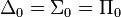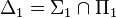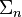# Difference between revisions of "User:IssaRice/Computability and logic/Some important distinctions and equivalences in introductory mathematical logic"

This page lists some important distinctions in introductory mathematical logic and computability theory. Bizarrely, most books won't even mention these distinctions, so you will probably be very confused at the start as you inevitably conflate these ideas.

• completeness: semantically complete (complete logic; the topic of the completeness theorem) vs negation-complete (complete theory; the topic of the first incompleteness theorem)
• decides: deciding a sentence vs a theory being decidable vs deciding every sentence vs a logic being decidable (decidability of logic has several equivalent formulations; this is the topic of the Entscheidungsproblem)
• soundness: sound logic (soundness theorem) vs sound theory
• truth in all interpretations (validity) vs truth in the intended interpretation (natural reading, standard interpretation)
• structure vs interpretation vs model
• interpretation: there's interpretation in the "structure" sense and also interpretation in the sense of embedding one theory inside another theory (e.g. doing arithmetic with sets)
• theory vs axioms
• language vs logic vs formal system vs system
• proves logically vs proves in a particular theory
•$\models$: when a set of sentences comes before the symbol vs when a structure comes before the symbol
• deciding (corresponding to computable total functions) vs recognizing (semi-deciding, computably enumerating; corresponding to computable partial functions)
• algorithm vs program vs index vs Godel number
• algorithm vs function computed by algorithm
• expresses vs captures (strong capture, weak capture)
• primitive recursive vs (general, μ) recursive
•$\Delta_0 = \Sigma_0 = \Pi_0$ vs$\Delta_1 = \Sigma_1 \cap \Pi_1$
•$\Sigma_n$ formulas vs relations vs sets
• enumerating vs computably enumerating

More speculative:

• different kinds of diagonalization?

There are also some important equivalences to keep in mind:

• Turing computable = computable = recursive = unbounded loops
• Primitive recursive = bounded loops
• computably enumerating = semi-deciding
• computably enumerating in order = deciding
• diagonalization lemma = rogers fixed point theorem
• Kleene's T predicate, godel beta function, Prf(m,n)